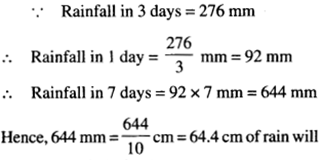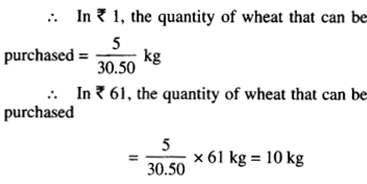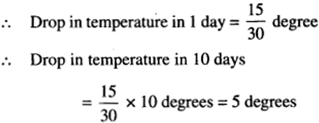NCERT Solutions for Class 6 Maths Chapter 12 Ratio and Proportion Ex 12.3 are part of NCERT Solutions for Class 6 Maths. Here we have given NCERT Solutions for Class 6 Maths Chapter 12 Ratio and Proportion Ex 12.3.

 Board CBSE Textbook NCERT Class Class 6 Subject Maths Chapter Chapter 12 Chapter Name Ratio and Proportion Exercise Ex 12.3 Number of Questions Solved 11 Category NCERT Solutions

## NCERT Solutions for Class 6 Maths Chapter 12 Ratio and Proportion Ex 12.3

Question 1.
If the cost of 7 m of cloth is ₹294, find the cost of 5 m of cloth.
Solution :Hence, the cost of 5 m of cloth is ₹ 210.

Question 2.
Ekta earns ₹ 1500 in 10 days. How much will she earn in 30 days?
Solution :Hence, Ekta will earn ₹ 4500 in 30 days.

Question 3.
If it has rained 276 mm in the last 3 days, how many cm of rain will fall in one full week (7 days)? Assume that the rain continues to fall at the same rate.
Solution :Hence, 644 mm = cm = 64.4 cm of rain will fall in one full week (7 days).

Question 4.
Cost of 5 kg of wheat is ₹30.50.
(a) What will be the cost of 8 kg of wheat?
(b) What quantity of wheat can be purchased in ₹ 61?
Solution :
(a) Cost of 5 kg of wheat = ₹ 30.50
∴ Cost of 1 kg of wheatHence, the cost of 8 kg of wheat will be ₹ 48.80
(b) In ₹ 30.50, the quantity of wheat that can be purchased = 5 kgHence, 10 kg of wheat can be purchased in

Question 5.
The temperature dropped 15 degree Celsius in the last 30 days. If the rate of temperature drop remains the same, how many degrees will the temperature drop in the next ten days?
Solution :
∴ Drop in temperature in 30 days =15 degreesHence, the temperature will drop 5 degrees in the next ten days.

Question 6.
Shaina pays ₹ 7500 as rent for 3 months. How much does she have to pay for a whole year, if the rent per month remains same₹
Solution :
∴ 1 Year = 12 months Y
∴ Rent paid by Shaina for 3 months = ₹ 7500
∴ Rent paid by Shaina for 1 month
₹ $$\frac{ 7500 }{ 3 }$$ = ₹ 2500
∴ Rent paid by Shaina for 12 months = ₹ (2500 x 12) = ₹ 30,000
Hence, Shaina will have to pay ₹ 30,000 for a whole year.

Question 7.
Cost of 4 dozens of bananas is ₹ 60. How many bananas can be purchased for ₹ 12.50?
Solution :
1 dozen = 12 items
∴ 4 dozens =12 x 4 items = 48 items
∴ Number of bananas that can be purchased for ₹60 = 48
∴ Number of bananas that can be pruchased for ₹ 1 = $$\frac{ 48 }{ 60 }$$
∴ Number of bananas that can be pruchased for ₹ 12.50 = $$\frac{ 48 }{ 60 }$$ × 12.50 = 10
Hence, 10 bananas can be purchased for ₹ 12.50.

Question 8.
The weight of 72 books is 9 kg. What is the weight of 40 such books?
Solution :
Weight of 72 books = 9 kg
∴ Weight of 1 books = $$\frac{ 9 }{ 72 }$$ kg  = $$\frac{ 1 }{ 8 }$$ kg
∴ Weight of 40 books = $$\frac{ 1 }{ 8 }$$ kg ×40 kg = 5 kg
Hence, the weight of 4(f such books is 5 kg.

Question 9.
A truck requires 108 liters of diesel for covering a distance of594 km. How much diesel will be required by the truck to cover a distance of 1650 km₹
Solution :
∴ Diesel required for covering a distance of 594 km =108 liters
∴ Diesel required for covering a distance of
1 km = $$\frac{ 108 }{ 594 }$$ litre
∴ Diesel required for covering a distance of
1650 km = $$\frac{ 108 }{ 594 }$$ × 1650 litres = 300
Hence, 300 liters of diesel will be required by the truck to cover a distance of 1650 km.

Question 10.
Raju purchases 10 pens for ₹ 150 and Manish buys 7 pens for ₹ 84. Can you say who got the pens cheaper₹
Solution :
For Raju
∵ Cost of 10 pens = ₹ 150
∴ Cost of 1 pen = ₹ $$\frac{ 150 }{ 10 }$$ = ₹ 15

For Manish
∵ Cost of 7 pens = ₹ 84
∴ Cost of 1 pen = ₹ $$\frac{ 84 }{ 7 }$$ = ₹ 12 7
So, Manish got the pens cheaper.

Question 11.
Anish made 42 runs in 6 overs and Anup made 63 runs in 7 overs. Who made more runs per over?
Solution :
For Anish
∵ Runs made in 6 overs = 42
∴ Runs made per over = $$\frac{ 42 }{ 6 }$$ = 7

For Anup
∵ Runs made in 7 overs = 63
∴ Runs made per over = $$\frac{ 63 }{ 7 }$$ = 9
So, Anup made more runs per over.

We hope the NCERT Solutions for Class 6 Maths Chapter 12 Ratio and Proportion Ex 12.3 help you. If you have any query regarding NCERT Solutions for Class 6 Maths Chapter 12 Ratio and Proportion Ex 12.3, drop a comment below and we will get back to you at the earliest.